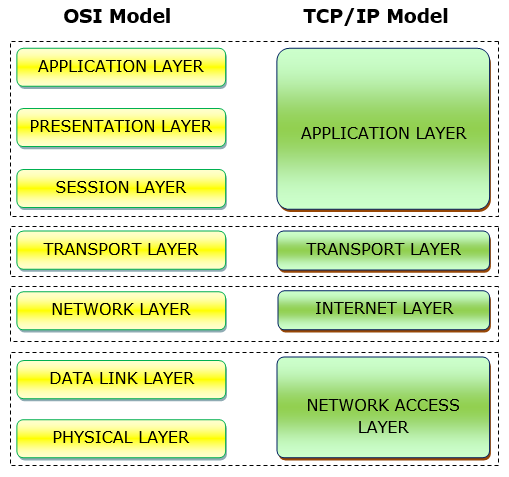# OSI vs. TCP/IP Reference Model

Computer NetworkComputer EngineeringMCA

## Similarities between OSI and TCP / IP Reference Models

• Both the reference models are based upon layered architecture.
• The layers in the models are compared with each other. The physical layer and the data link layer of the OSI model correspond to the link layer of the TCP/IP model. The network layers and the transport layers are the same in both the models. The session layer, the presentation layer and the application layer of the OSI model together form the application layer of the TCP/IP model.
• In both the models, protocols are defined in a layer-wise manner.
• In both models, data is divided into packets and each packet may take the individual route from the source to the destination.

## Differences between OSI and TCP / IP Reference Models

• OSI model is a generic model that is based upon functionalities of each layer. TCP/IP model is a protocol-oriented standard.
• OSI model distinguishes the three concepts, namely, services, interfaces, and protocols. TCP/IP does not have a clear distinction between these three.
• OSI model gives guidelines on how communication needs to be done, while TCP/IP protocols layout standards on which the Internet was developed. So, TCP/IP is a more practical model.
• In OSI, the model was developed first and then the protocols in each layer were developed. In the TCP/IP suite, the protocols were developed first and then the model was developed.
• The OSI has seven layers while the TCP/IP has four layers.

The following diagram shows the corresponding layers of OSI and TCP/IP models −